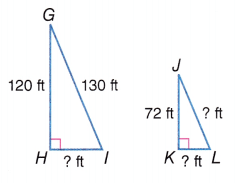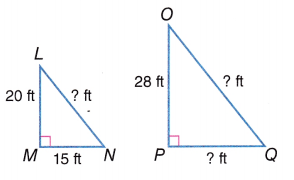# McGraw Hill Math Grade 8 Lesson 20.3 Answer Key Right Triangles and Pythagorean Theorem

Practice the questions of McGraw Hill Math Grade 8 Answer Key PDF Lesson 20.3 Right Triangles and Pythagorean Theorem to secure good marks & knowledge in the exams.

## McGraw-Hill Math Grade 8 Answer Key Lesson 20.3 Right Triangles and Pythagorean Theorem

Exercises

SOLVE

Use the Pythagorean Theorem to determine the length of the missing side.

Question 1.
If side A is 6 and side B is 8, then side C (the hypotenuse) is _____________
C = 10,

Explanation:
As side A is 6 and side B is 8, then side C (the hypotenuse) is applying Pythagorean theorem that is the square of the length of the hypotenuse of a right triangle equals the sum of the squares of the lengths of the other two sides. So C = square root of (A2 + B2 ) = square root of (62 + 82)= square root of (36 + 64) = square root of 100 = 10.

Question 2.
If side A is 9 and side B is 9, then side C (the hypotenuse) is _____________
C = 12.72,

Explanation:
As side A is 9 and side B is 9, then side C (the hypotenuse) is applying Pythagorean theorem that is the square of the length of the hypotenuse of a right triangle equals the sum of the squares of the lengths of the other two sides. So C = square root of (A2 + B2 ) = square root of (92 + 92)= square root of (81 + 81) = square root of 162 , approximately equal to 12.72.

Question 3.
If side A is 10 and side B is 24, then side C (the hypotenuse) is _____________
C = 26,

Explanation:
As side A is 10 and side B is 24, then side C (the hypotenuse) is applying Pythagorean theorem that is the square of the length of the hypotenuse of a right triangle equals the sum of the squares of the lengths of the other two sides. So C = square root of (A2 + B2 ) = square root of (102 + 242)= square root of (100 + 576) = square root of 676 = 26.

Question 4.
If side A is 9 and side C (the hypotenuse) is 15, then side B is _____________
B = 12,

Explanation:
As side A is 9 and side C (the hypotenuse) is 15, then side B is applying Pythagorean theorem that is the square of the length of the hypotenuse of a right triangle equals the sum of the squares of the lengths of the other two sides. C = square root of (A2 + B2 ) therefore B = square root of (C2 – A2)= square root of (225 – 81) = square root of 144 = 12.

Question 5.
If side B is 6 and the hypotenuse is 10, then side A is _____________
A = 8,

Explanation:
As side B is 6 and the hypotenuse is 10, then side A is applying Pythagorean theorem that is the square of the length of the hypotenuse of a right triangle equals the sum of the squares of the lengths of the other two sides. So C = square root of (A2 + B2 ) therefore A = square root of (C2 – B2)= square root of (100 – 36) = square root of 64 = 8.

Question 6.
If side A is 12 and side B is 5, then side C (the hypotenuse) is _____________
C = 13,

Explanation:
As side A is 12 and side B is 5, then side C (the hypotenuse) is applying Pythagorean theorem that is the square of the length of the hypotenuse of a right triangle equals the sum of the squares of the lengths of the other two sides. So C = square root of (A2 + B2 ) = square root of (122 + 52) = square root of (144 + 25) = square root of 169 = 13.

Find the missing sides of the following pairs of similar right triangles:

Question 1.
HI = ___________ ft
KL = ___________ ft
JL = ____________ ftHI = 50 ft.,
KL = 30 ft.,
JL =  78 ft.,

Explanation:
For finding HI we apply Pythagorean theorem that is the square of the length of the hypotenuse of a right triangle equals the sum of the squares of the lengths of the other two sides. So HI = square root of (GI2 – GH2 ) = square root of (1302 – 1202) = square root of (16,900 – 14,400) = square root of 2,500 = 50 ft.
Now finding KL let it be x as GHI and JKL are similar triangles that means the ratios of the sides are equal  HI/GH = KL/JK = 50/120 = x/72 cross multiplying for the unknown we get
120x = 50 X 72, x = 5 X 72/12 = 5 X 6 = 30 ft.
Now JL we apply Pythagorean theorem that is the square of the length of the hypotenuse of a right triangle equals the sum of the squares of the lengths of the other two sides. So HI = square root of (JK2 + KL2 ) = square root of (722 + 302) = square root of (5,184 + 900) = square root of 6,084 = 78 ft.

Question 2.
LN = ___________ m
PQ = ___________ m
OQ = ____________ mLN = 25 ft., = 7.62 m,
PQ = 21 ft., = 6.4008 m,
OQ = 35 ft., = 10.668 m,

Explanation:
For finding LN we apply Pythagorean theorem that is the square of the length of the hypotenuse of a right triangle equals the sum of the squares of the lengths of the other two sides. So LN = square root of (LM2 + MN2 ) = square root of (202 + 152) = square root of (400 + 225) = square root of 625 = 25 ft. As 1 foot is equal to 0.3048 meter,
so 25 X 0.3048 m = 7.62 m.
Now finding PQ let it be x as LMN and OPQ are similar triangles that means the ratios of the sides are equal  MN/LM = PQ/OP = 15/20 = x/28 cross multiplying for the unknown we get
20x = 15 X 28, x = 3 X 28/4 = 3 X 7 = 21 ft., = 21 X 0.3048 m = 6.4008 m.
Now OQ we apply Pythagorean theorem that is the square of the length of the hypotenuse of a right triangle equals the sum of the squares of the lengths of the other two sides. So OQ = square root of (OP2 + PQ2 ) = square root of (282 + 212) = square root of (784 + 441) = square root of 1,225 = 35 ft., = 35 X 0.3048 m =  10.668 m.

Question 3.
RT = ___________ in.
VW = ___________ in.
UW = ____________ in.RT = 7.071 ft., = 84.852 in.,
VW = 20 ft., = 240 in.,
UW = 28.284 ft., = 339.400 in.,

Explanation:
For finding RT we apply Pythagorean theorem that is the square of the length of the hypotenuse of a right triangle equals the sum of the squares of the lengths of the other two sides. So HI = square root of (RS2 + ST2 ) = square root of (52 + 52) = square root of (25 + 25) = square root of 50 = 7.071 ft. As 1 foot is equal to 12 inch we get 7.071 X 12 inch = 84.852 in, Now finding VW let it be x as RST and UVW are similar triangles that means the ratios of the sides are equal  ST/RS = VW/UV = 5/5 = x/20 cross multiplying for the unknown we get 5x = 5 X 20, x = 20 ft., = 20 X 12 in = 240 in.,
Now UW we apply Pythagorean theorem that is the square of the length of the hypotenuse of a right triangle equals the sum of the squares of the lengths of the other two sides. So UW = square root of (UV2 + VW2 ) = square root of (202 + 202) = square root of (400 + 400) = square root of 800 = 28.284 ft., = 339.408 in.

Question 4.
If the two triangles are similar, then what is the length of the missing side?